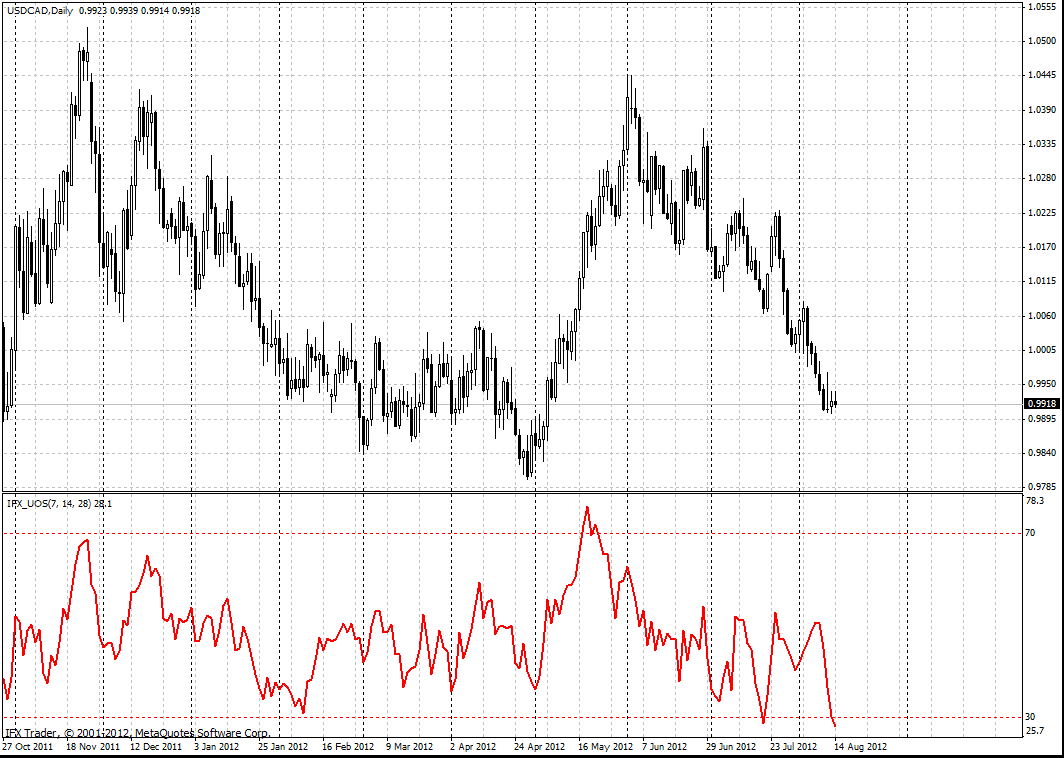# 终极波动指标

#### 计算

TL (i) = MIN (LOW (i) || CLOSE (i - 1))

BP (i) = CLOSE (i) - TL (i)

TR (i) = MAX (HIGH (i) - LOW (i) || HIGH (i) - CLOSE (i - 1) || CLOSE (i - 1) - LOW (i))

BPSUM (N) = SUM (BP (i), i)

TRSUM (N) = SUM (TR (i), i)

RawUO = 4 * (BPSUM (1) / TRSUM (1)) + 2 * (BPSUM (2) / TRSUM (2)) + (BPSUM (3) / TRSUM (3))

UO = ( RawUO / (4 + 2 + 1)) * 100, where

MIN - 最小读数;

MAX - 最大读数;

|| - logical OR;

LOW (i) - 当前栏的最低价格;

HIGH (i) - 当前栏的最高价格;

CLOSE (i) - 当前栏的收盘价;

CLOSE (i - 1) - 先前栏的收盘价;

TL (i) - 真低;

BP (i) - 购买压力;

TR (i) - 真实范围;

BPSUM (N) - BP读数为N时间段的总和（N等于1，i=7栏;当N= 2，I =14栏，当N = 3，= 28栏）;

TRSUM (N) - TR读数为N时间段的总和（N等于1，i=7栏;当N= 2，I =14栏，当N = 3，= 28栏）;

RawUO - 原始终极波动指标读数;

UO - 终极波动指标读数.

#### 交易使用

• 如果出现看跌背离其中价格的增长和指标数值低于50，有必要开空头头寸。在价格跌破30水平以下的情况下，关闭空头头寸;
• 如果出现看涨收敛其中价格下降及指标高于50以上，开多头头寸 。价格克服了70时，关闭多头头寸 。#### InstaForex终极波动指标参数

fastperiod = 7

middleperiod = 14

slowperiod = 28

fastK = 4

middleK = 2

slowK = 1